# DHARMa: residual diagnostics for hierarchical (multi-level/mixed) regression models

#### 2019-03-05

Abstract

The ‘DHARMa’ package uses a simulation-based approach to create readily interpretable scaled (quantile) residuals for fitted (generalized) linear mixed models. Currently supported are linear and generalized linear (mixed) models from ‘lme4’ (classes ‘lmerMod’, ‘glmerMod’), ‘glmmTMB’ and ‘spaMM’, generalized additive models (‘gam’ from ‘mgcv’), ‘glm’ (including ‘negbin’ from ‘MASS’, but excluding quasi-distributions) and ‘lm’ model classes. Moreover, externally created simulations, e.g. posterior predictive simulations from Bayesian software such as ‘JAGS’, ‘STAN’, or ‘BUGS’ can be processed as well. The resulting residuals are standardized to values between 0 and 1 and can be interpreted as intuitively as residuals from a linear regression. The package also provides a number of plot and test functions for typical model misspecification problems, such as over/underdispersion, zero-inflation, and residual spatial and temporal autocorrelation.

# Motivation

Residual interpretation for generalized linear mixed models (GLMMs) is often problematic. As an example, here two Poisson GLMMs, one that is lacking a quadratic effect, and one that fits the data perfectly. I show three standard residuals diagnostics each. Which is the misspecified model?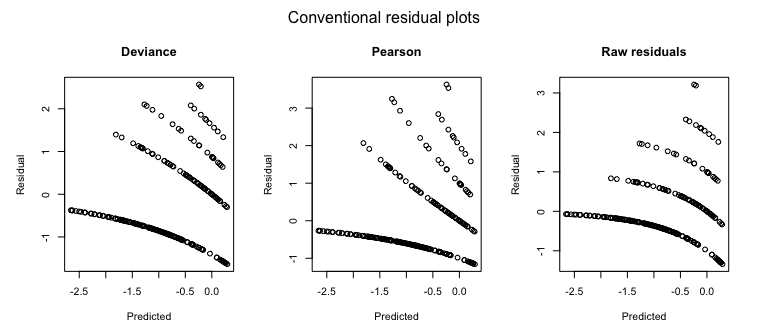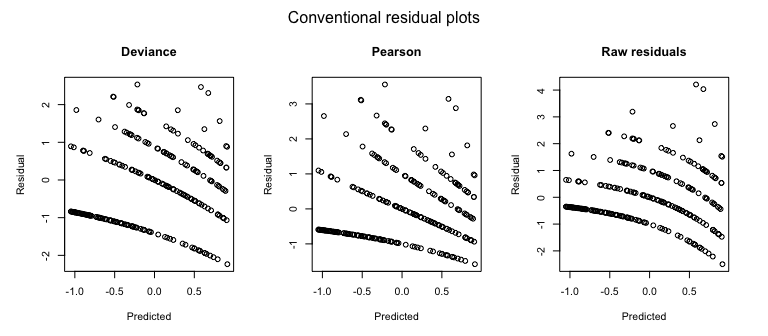Just for completeness - it was the first one. But don’t get too excited if you got it right. Either you were lucky, or you noted that the first model seems a bit overdispersed (range of the Pearson residuals). But even when noting that, would you have added a quadratic effect, instead of adding an overdispersion correction? The point here is that misspecifications in GL(M)Ms cannot reliably be diagnosed with standard residual plots, and GLMMs are thus often not as thoroughly checked as LMs.

One reason why GL(M)Ms residuals are harder to interpret is that the expected distribution of the data changes with the fitted values. Reweighting with the expected variance, as done in Pearson residuals, or using deviance residuals, helps a bit, but does not lead to visually homogenous residuals even if the model is correctly specified. As a result, standard residual plots, when interpreted in the same way as for linear models, seem to show all kind of problems, such as non-normality, heteroscedasticity, even if the model is correctly specified. Questions on the R mailing lists and forums show that practitioners are regularly confused about whether such patterns in GL(M)M residuals are a problem or not.

But even experienced statistical analysts currently have few options to diagnose misspecification problems in GLMMs. In my experience, the current standard practice is to eyeball the residual plots for major misspecifications, potentially have a look at the random effect distribution, and then run a test for overdispersion, which is usually positive, after which the model is modified towards an overdispersed / zero-inflated distribution. This approach, however, has a number of problems, notably:

• Overdispersion often comes from missing or misspecified predictors. Standard residual plots make it difficult to test for residual patterns against the predictors to check for candidates.

• Not all overdispersion is the same. For count data, the negative binomial creates a different distribution than adding observation-level random effects to the Poisson. Once overdispersion is corrected, such violations of distributional assumptions are not detectable with standard overdispersion tests (because the tests only looks at total dispersion), and nearly impossible to see visually from standard residual plots.

• Dispersion frequently varies with predictors (heteroscedasticity). This can have a significant effect on the inference. While it is standard to tests for heteroscedasticity in linear regressions, heteroscedasticity is currently hardly ever tested for in GLMMs, although it is likely as frequent and influential.

• Moreover, if residuals are checked, they are usually checked conditional on the fitted random effect estimates. Thus, standard checks only check the final level of the random structure in a GLMM. One can perform extra checks on the random effects, but it is somewhat unsatisfactory that there is no check on the entire model structure.

DHARMa aims at solving these problems by creating readily interpretable residuals for generalized linear (mixed) models that are standardized to values between 0 and 1, and that can be interpreted as intuitively as residuals for the linear model. This is achieved by a simulation-based approach, similar to the Bayesian p-value or the parametric bootstrap, that transforms the residuals to a standardized scale. The basic steps are:

1. Simulate new data from the fitted model for each observation.

2. For each observation, calculate the empirical cumulative density function for the simulated observations, which describes the possible values (and their probability) at the predictor combination of the observed value, assuming the fitted model is correct.

3. The residual is then defined as the value of the empirical density function at the value of the observed data, so a residual of 0 means that all simulated values are larger than the observed value, and a residual of 0.5 means half of the simulated values are larger than the observed value.

These steps are visualized in the following figure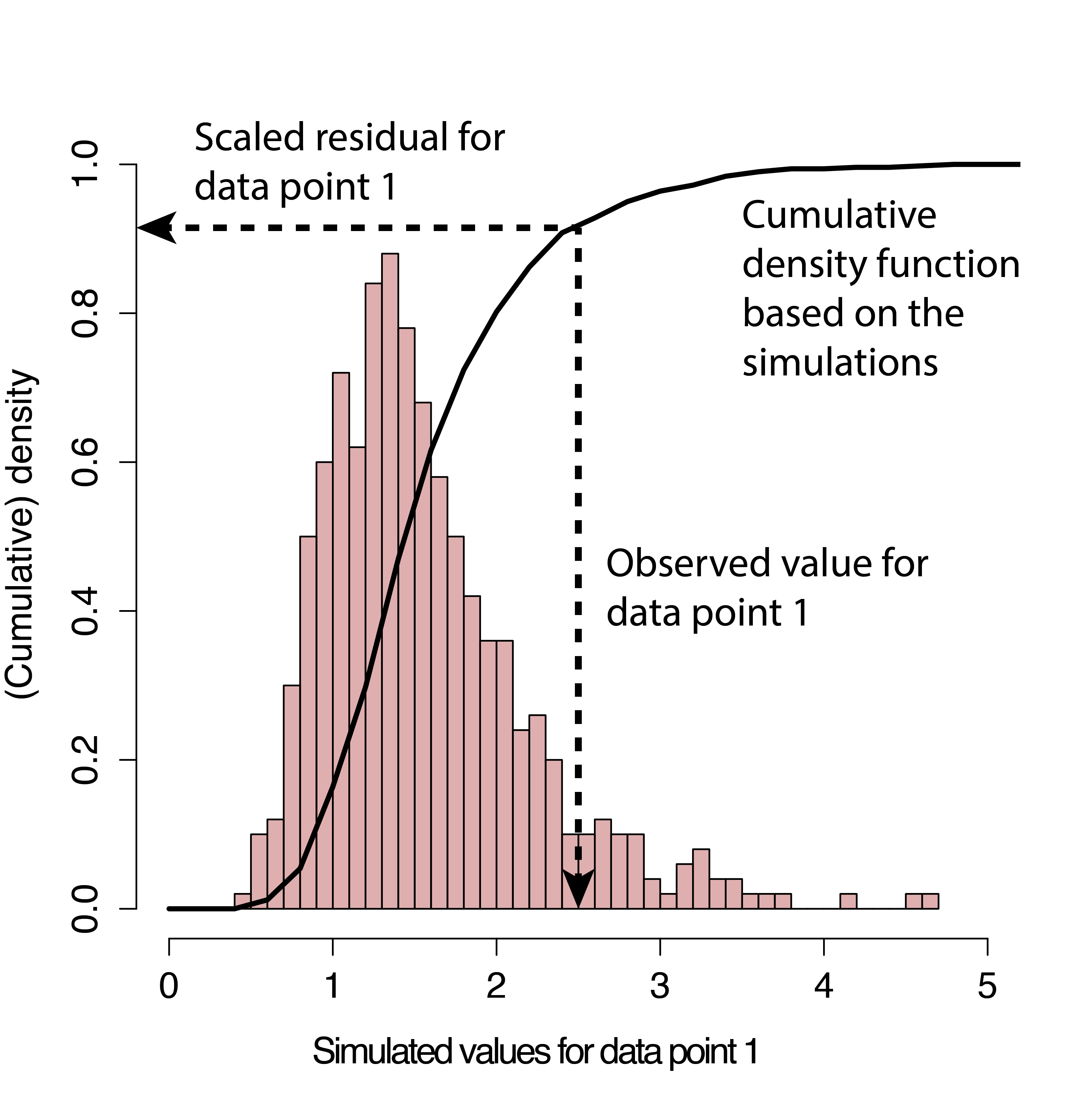The key motivation behind this definition is that we have a clear expectations how these residuals should be distributed. If the model is correctly specified, then the observed data should look as if they were created from the fitted model. Hence, for a correctly specified model, all values of the cumulative distribution should appear with equal probability. That means we expect the distribution of the residuals to be flat, regardless of the model structure (Poisson, binomial, random effects and so on).

I currently prepare a more exact statistical justification for the approach in an accompanying paper, but if you must provide a reference in the meantime I would suggest citing

• Dunn, K. P., and Smyth, G. K. (1996). Randomized quantile residuals. Journal of Computational and Graphical Statistics 5, 1-10.

• Gelman, A. & Hill, J. Data analysis using regression and multilevel/hierarchical models Cambridge University Press, 2006

p.s.: DHARMa stands for “Diagnostics for HierArchical Regression Models” – which, strictly speaking, would make DHARM. But in German, Darm means intestines; plus, the meaning of DHARMa in Hinduism makes the current abbreviation so much more suitable for a package that tests whether your model is in harmony with your data:

From Wikipedia, 28/08/16: In Hinduism, dharma signifies behaviours that are considered to be in accord with rta, the order that makes life and universe possible, and includes duties, rights, laws, conduct, virtues and ‘‘right way of living’’.

# Workflow in DHARMa

If you haven’t installed the package yet, either run

``install.packages("DHARMa")``

Or follow the instructions on https://github.com/florianhartig/DHARMa to install a development version.

``````library(DHARMa)
citation("DHARMa")``````
``````##
## To cite package 'DHARMa' in publications use:
##
##   Florian Hartig (2019). DHARMa: Residual Diagnostics for
##   Hierarchical (Multi-Level / Mixed) Regression Models. R package
##   version 0.2.4. http://florianhartig.github.io/DHARMa/
##
## A BibTeX entry for LaTeX users is
##
##   @Manual{,
##     title = {DHARMa: Residual Diagnostics for Hierarchical (Multi-Level / Mixed) Regression Models},
##     author = {Florian Hartig},
##     year = {2019},
##     note = {R package version 0.2.4},
##     url = {http://florianhartig.github.io/DHARMa/},
##   }``````

## Calculating scaled residuals

The scaled (quantile) residuals are calculated with the simulateResiduals() function. The default number of simulations to run is 250, which proved to be a reasonable compromise between computation time and precision, but if high precision is desired, n should be raised to 1000 at least.

``simulationOutput <- simulateResiduals(fittedModel = fittedModel, n = 250)``

What the function does is a) creating n new synthetic datasets by simulating from the fitted model, b) calculates the cumulative distribution of simulated values for each observed value, and c) returning the quantile value that corresponds to the observed value.

For example, a scaled residual value of 0.5 means that half of the simulated data are higher than the observed value, and half of them lower. A value of 0.99 would mean that nearly all simulated data are lower than the observed value. The minimum/maximum values for the residuals are 0 and 1.

The calculated residuals are stored in

``simulationOutput\$scaledResiduals``

As discussed above, for a correctly specified model we would expect

• a uniform (flat) distribution of the overall residuals

• uniformity in y direction if we plot against any predictor.

Note: the expected uniform distribution is the only differences to the linear regression that one has to keep in mind when interpreting DHARMa residuals. If you cannot get used to this and you must have residuals that behave exactly like a linear regression, you can access a normal transformation of the residuals via

``simulationOutput\$scaledResidualsNormal``

These normal residuals will behave exactly like the residuals of a linear regression. However, for reasons of a) numeric stability with low number of simulations and b) my conviction that it is much easier to visually detect deviations from uniformity than normality, I would STRONGLY advice against using this transformation.

## Plotting the scaled residuals

We can get a visual impression of these properties with the plot.DHARMa() function

``plot(simulationOutput)``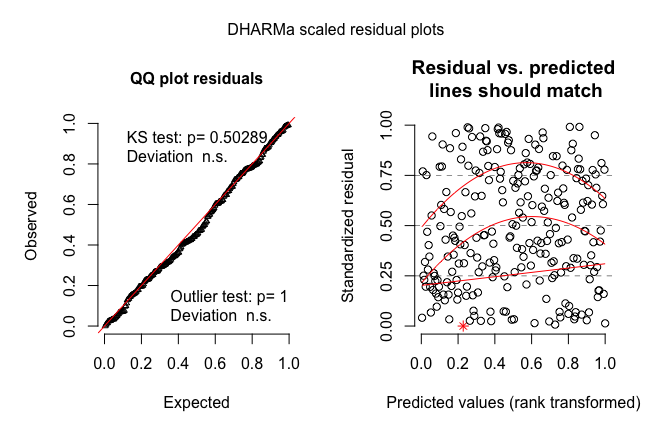which creates a qq-plot to detect overall deviations from the expected distribution (with added tests for uniformity and outliers), and a plot of the residuals against the predicted value.

In the plot res ~ predicted, simulation outliers are highlighted as red stars. By simulation outliers, I mean data points that are outside the range of simulated values. Because no data was simulated in that range, we actually don’t know “how much” these values deviate from the model expecation, so the term “outlier” should be used with a grain of salt. Note also that the probability of an outlier depends on the number of simulations (in fact, it is 1/(nSim +1) for each side), so whether the existence of outliers is a reason for concern depends also on the number of simulations.

To provide a visual aid in detecting deviations from uniformity in y-direction, the plot of the residuals against the predicted values also performs an (optional) quantile regression, which provides 0.25, 0.5 and 0.75 quantile lines across the plots. These lines should be straight, horizontal, and at y-values of 0.25, 0.5 and 0.75. Note, however, that some deviations from this are to be expected by chance, even for a perfect model, especially if the sample size is small.
The quantile regression can be very slow for large datasets. You can chose to use a simpler method with the option quantreg = F.

If you want to plot the residuals against other predictors (highly recommend), you can use the function

``plotResiduals(YOURPREDICTOR, simulationOutput\$scaledResiduals)``

which does the same quantile plot as the main plotting function.

## Formal goodness-of-fit tests on the scaled residuals

To support the visual inspection of the residuals, the DHARMa package provides a number of specialized goodness-of-fit tests on the simulated residuals:

• testUniformity()
• testOutliers()
• testDispersion()
• testZeroinflation()
• testGeneric()
• testTemporalAutocorrelation()
• testSpatialAutocorrelation()

that basically do what they say. See the help of the functions and further comments below for a more detailed description. The wrapper function testResiduals calculates the first three tests, including their graphical outputs

``testResiduals(simulationOutput)``

## Simulation options

There are a few important technical details regarding how the simulations are performed, in particular regarding the treatments of random effects and integer responses. It is strongly recommended to read the help of

``?simulateResiduals``

#### Refit

``simulationOutput <- simulateResiduals(fittedModel = fittedModel, refit = T)``
• if refit = F (default), new data is simulated from the fitted model, and residuals are calculated by comparing the observed data to the new data

• if refit = T, a parametric bootstrap is performed, meaning that the model is refit to the new data, and residuals are created by comparing observed residuals against refitted residuals

The second option is much much slower, and also seemed to have lower power in some tests I ran. ** It is therefore not recommended for standard residual diagnostics!** I only recommend using it if you know what you are doing, and have particular reasons, for example if you estimate that the tested model is biased. A bias could, for example, arise in small data situations, or when estimating models with shrinkage estimators that include a purposeful bias, such as ridge/lasso, random effects or the splines in GAMs. My idea was then that simulated data would not fit to the observations, but that residuals for model fits on simulated data would have the same patterns/bias than model fits on the observed data.

Note also that refit = T can sometimes run into numerical problems, if the fitted model does not converge on the newly simulated data.

#### Random effect simulations

The second option is the treatment of the stochastic hierarchy. In a hierarchical model, several layers of stochasticity are placed on top of each other. Specifically, in a GLMM, we have a lower level stochastic process (random effect), whose result enters into a higher level (e.g. Poisson distribution). For other hierarchical models, such as state-space models, similar considerations apply, but the hierarchy can be more complex. When simulating, we have to decide if we want to re-simulate all stochastic levels, or only a subset of those. For example, in a GLMM, it is common to only simulate the last stochastic level (e.g. Poisson) conditional on the fitted random effects, meaning that the random effects are set on the fitted values.

For controlling how many levels should be re-simulated, the simulateResidual function allows to pass on parameters to the simulate function of the fitted model object. Please refer to the help of the different simulate functions (e.g. ?simulate.merMod) for details. For merMod (lme4) model objects, the relevant parameters are “use.u”, and “re.form”, as, e.g., in

``simulationOutput <- simulateResiduals(fittedModel = fittedModel, n = 250, use.u = T)``

If the model is correctly specified and the fitting procedure is unbiased (disclaimer: GLMM estimators are not always unbiased), the simulated residuals should be flat regardless how many hierarchical levels we re-simulate. The most thorough procedure would be therefore to test all possible options. If testing only one option, I would recommend to re-simulate all levels, because this essentially tests the model structure as a whole. This is the default setting in the DHARMa package. A potential drawback is that re-simulating the random effects creates more variability, which may reduce power for detecting problems in the upper-level stochastic processes.

#### Integer treatment / randomization

A third option is the treatment of integer responses. The background of this option is that, for integer-valued variables, some additional steps are neccessary to make sure that the residual distribution becomes flat (essentially, we have to smoothen away the integer nature of the data). The idea is explained in

• Dunn, K. P., and Smyth, G. K. (1996). Randomized quantile residuals. Journal of Computational and Graphical Statistics 5, 1-10.

The simulateResiduals function will automatically check if the family is integer valued, and apply randomization if that is the case. I see no reason why one would not want to randomize for an integer-valued function, so the parameter should usually not be changed.

#### Calculating residuals per group

In many situations, it can be useful to look at residuals per group, e.g. to see how much the model over / underpredicts per plot, year or subject. To do this, use the recalculateResiduals() function, together with a grouping variable

``simulationOutput = recalculateResiduals(simulationOutput, group = testData\$group)``

you can keep using the simulation output as before. Note, hover, that items such as simulationOutput\$scaledResiduals now have as many entries as you have groups, so if you perform plots by hand, you have to aggregate predictors in the same way. For the latter purpose, recalculateResiduals adds a function aggregateByGroup to the output.

#### Reproducibility notes, random seed and random state

As DHARMa uses simulations to calculate the residuals, a naive implementation of the algorithm would mean that residuals would look slightly different each time a DHARMa calculation is executed. This might both be confusing and bear the danger that a user would run the simulation several times and take the result that looks better (which would amount to multiple testing / p-hacking).

By default, DHARMa therefore fixes the random seed to the same value every time a simulation is run, and afterwards restores the random state to the old value. This means that you will get exactly the same residual plot each time. If you want to avoid this behavior, for example for simulation experiments on DHARMa, use seed = NULL -> no seed set, but random state will be restored, or seed = F -> no seed set, and random state will not be restored. Whether or not you fix the seed, the setting for the random seed and the random state are stored in

``simulationOutput\$randomState``

If you want to reproduce simualtions for such a run, set the variable .Random.seed by hand, and simulate with seed = NULL.

Moreover (general advice), to ensure reproducibility, it’s advisable to add a set.seed() at the beginning, and a session.info() at the end of your script. The latter will lists the version number of R and all loaded packages.

# Interpreting residuals and recognizing misspecification problems

In all plots / tests that were shown so far, the model was correctly specified, resulting in “perfect” residual plots. In this section, we discuss how to recognize and interpret model misspecifications in the scaled residuals. Note, however, that

1. The fact that none of the here-presented tests shows a misspecification problem doesn’t proof that the model is correctly specified. There are likely a large number of structural problems that will not show a pattern in the standard residual plots.

2. Conversely, while a clear pattern in the residuals indicates with good reliability that the observed data would not be likely to originate from the fitted model, it doesn’t neccessarily indicate that the model results are not usuable. There are many cases where it is common practice to work “wrong models”. For example, random effect estimates (in particular in GLMMs) are often slightly biased, especially if the model is fit with MLE. For that reason, DHARMa will often show a slight pattern in the residuals even if the model is correctly specified, and tests for this can get significant for large sample sizes. Another example is data that is missing at random (MAR) (see here). It is know that this phenomenon does not createa bias on the fixed effect estimates, and it is therefore common practice to fit this data with mixed models. Nevertheless, DHARMa recognizes that the observed data looks different than what would be expected from the model assummptions, and flags the model as problemaetic

Important conclusion: DHARMa only flags a difference between the observed and expected data - the user has to decide whether this difference is actually a problem for the analysis!

## Overdispersion / underdispersion

The most common concern for GLMMs is overdispersion, underdispersion and zero-inflation.

Over/underdispersion refers to the phenomenon that residual variance is larger/smaller than expected under the fitted model. Over/underdispersion can appear for any distributional family with fixed variance, in particular for Poisson and binomial models.

A few general rules of thumb

• You can detect overdispersion / zero-inflation only AFTER fitting the model
• Overdispersion is more common than underdispersion
• If overdispersion is present, confidence intervals tend to be too narrow, and p-values to small. The opposite is true for underdispersion
• A common reason for overdispersion is a misspecified model. When overdispersion is detected, one should therefore first search for problems in the model specification (e.g. by plotting residuals against predictors with DHARMa), and only if this doesn’t lead to success, overdispersion corrections such as individual-level random effects or changes in the distribution should be applied

#### An example of overdispersion

This this is how overdispersion looks like in the DHARMa residuals

``````testData = createData(sampleSize = 500, overdispersion = 2, family = poisson())
fittedModel <- glmer(observedResponse ~ Environment1 + (1|group) , family = "poisson", data = testData)

simulationOutput <- simulateResiduals(fittedModel = fittedModel)
plot(simulationOutput)``````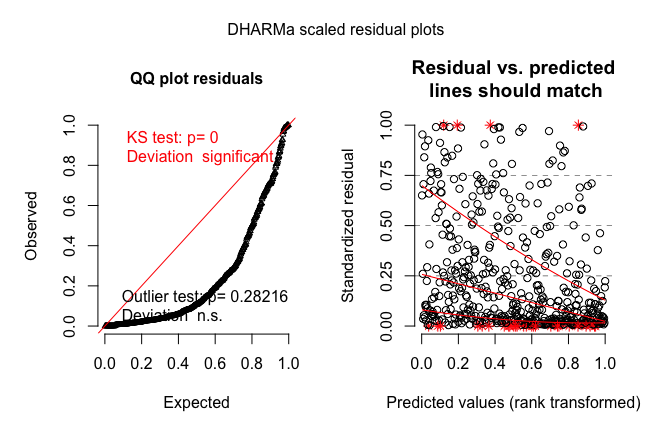Note that we get more residuals around 0 and 1, which means that more residuals are in the tail of distribution than would be expected under the fitted model.

#### An example of underdispersion

This is an example of underdispersion

``````testData = createData(sampleSize = 500, intercept=0, fixedEffects = 2, overdispersion = 0, family = poisson(), roundPoissonVariance = 0.001, randomEffectVariance = 0)
fittedModel <- glmer(observedResponse ~ Environment1 + (1|group) , family = "poisson", data = testData)

summary(fittedModel)``````
``````## Generalized linear mixed model fit by maximum likelihood (Laplace
##   Approximation) [glmerMod]
##  Family: poisson  ( log )
## Formula: observedResponse ~ Environment1 + (1 | group)
##    Data: testData
##
##      AIC      BIC   logLik deviance df.resid
##   1031.1   1043.8   -512.6   1025.1      497
##
## Scaled residuals:
##      Min       1Q   Median       3Q      Max
## -0.64083 -0.35390 -0.05813  0.22834  0.91703
##
## Random effects:
##  Groups Name        Variance Std.Dev.
##  group  (Intercept) 0        0
## Number of obs: 500, groups:  group, 10
##
## Fixed effects:
##              Estimate Std. Error z value Pr(>|z|)
## (Intercept)  -0.13024    0.05831  -2.233   0.0255 *
## Environment1  2.19567    0.08519  25.772   <2e-16 ***
## ---
## Signif. codes:  0 '***' 0.001 '**' 0.01 '*' 0.05 '.' 0.1 ' ' 1
##
## Correlation of Fixed Effects:
##             (Intr)
## Environmnt1 -0.818
## convergence code: 0
## singular fit``````
``````# plotConventionalResiduals(fittedModel)

simulationOutput <- simulateResiduals(fittedModel = fittedModel)
plot(simulationOutput)``````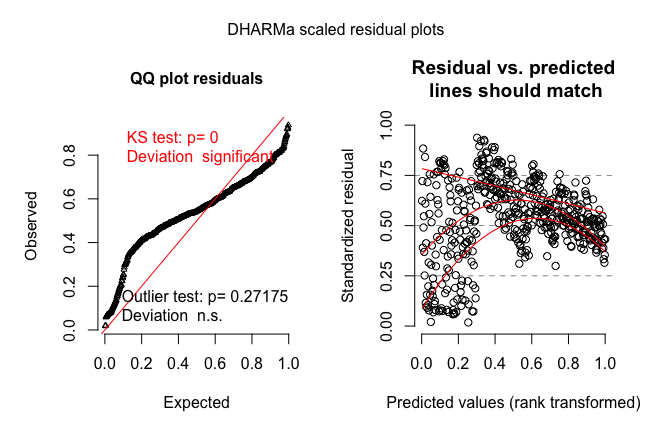``testUniformity(simulationOutput = simulationOutput)``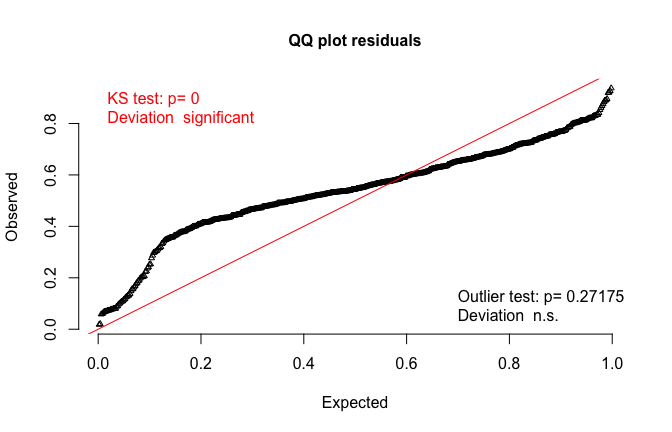``````##
##  One-sample Kolmogorov-Smirnov test
##
## data:  simulationOutput\$scaledResiduals
## D = 0.21859, p-value < 2.2e-16
## alternative hypothesis: two-sided``````

Here, we get too many residuals around 0.5, which means that we are not getting as many residuals as we would expect in the tail of the distribution than expected from the fitted model.

#### Testing for over/underdispersion

Although, as discussed above, over/underdispersion will show up in the residuals, and it’s possible to detect it with the testUniformity function, simulations show that this test is less powerful than more targeted tests.

DHARMa therefore contains two overdispersion tests that compares the dispersion of simulated residuals to the observed residuals.

1. A non-parametric test on the simulated residuals
2. A non-parametric overdispersion test on the re-fitted residuals.

You can call these tests as follows:

``````# Option 2
testDispersion(simulationOutput)``````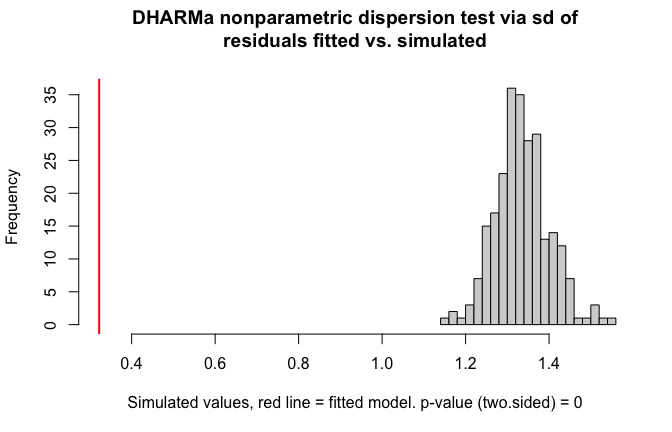``````##
##  DHARMa nonparametric dispersion test via sd of residuals fitted
##  vs. simulated
##
## data:  simulationOutput
## ratioObsSim = 0.24135, p-value < 2.2e-16
## alternative hypothesis: two.sided``````
``````# Option 3
simulationOutput2 <- simulateResiduals(fittedModel = fittedModel, refit = T, n = 20)
testDispersion(simulationOutput2)``````